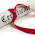In this article I am going to draw line with Bresenham's line algorithm. Find more about Bresenham's line algorithm at http://en.wikipedia.org/wiki/Bresenham's_line_algorithm

### CSS :

```#line b {
position:absolute;
color:rgb(91,111,135);
}

#line {
position:relative;
margin:auto;
width:400px;
height:400px;
vertical-align:center;
}

```

### jQuery :

x1, y1 : First point coordinates
x2, y2 : Second point coordinates
dx : Difference in X axis coordinates of two points
dy : Difference in Y axis coordinates of two points
p : Variable to calculate increments in X and Y coordinates

```var x1=10, x2=400, y1=10, y2=400;
var dx=x2 - x1, dy=y2 - y1;
var p=2*dy - dx, i=dx;
while (i > 0) {
putpixel(x1, y1);
if (p < 0) {
x1=x1 + 1;
p=p + 2*dy;
} else {
x1=x1 + 1;
y1=y1 + 1;
p=p + 2*dy - 2*dx;
}
i--;
}

function putpixel(mx, my) {
\$("#line").append("<b style='left:" + mx + "px;top:" + my + "px'>.</b>");
}

```

1.Your blogs are great.Are you also searching for nursing pico writing help? we are the best solution for you. We are best known for delivering nursing writing services to students without having to break the bank.

2.## BlogrollSrinivas Dasari find me on facebook follow me on twitter